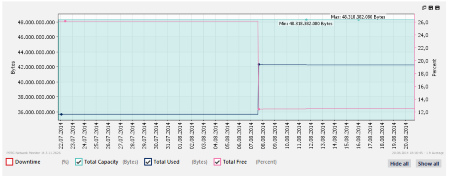### What is this?

This knowledgebase contains questions and answers about PRTG Network Monitor and network monitoring in general.Intuitive to Use. Easy to manage.
More than 500,000 users rely on Paessler PRTG every day. Find out how you can reduce cost, increase QoS and ease planning, as well.

View all Tags

# How can I monitor capacity and used disk space on a NetApp?

#### 0

The SNMP NetApp Disk Free sensor only shows free disk space in percent and absolute values. Additionally, I would like to retrieve and show the maximum capacity of NetApp volumes, respectively of the whole NetApp storage system, and compare it to the disk space that is actually used. How can I achieve this?

Last change on Jul 18, 2019 10:36:58 AM by

# Monitoring the total capacity of a NetApp

The SNMP NetApp Disk Free sensor monitors free space on a NetApp storage system. It shows, among other things, free space in percent and bytes channels in one dedicated sensor for each selected volume, but ignores the total capacity of a disk because this value usually does not change.

## Calculating the capacity of a NetApp volume

However, if you need the maximum capacity value, you can calculate the total disk space with the existing channels of your NetApp Disk Free sensors. Actually, PRTG already retrieves the total disk space value from a NetApp disk to calculate the values for the free space and bytes channels:

```Channel0 “Free Space”:
100 * AvailableSpace / TotalCapacity [Percent]

Channel1 “Free Bytes”:
AvailableSpace * 1024 [Bytes]
```

To get the capacity of a disk, divide the free space in bytes of Channel1 by the free space in percent of Channel0. So you can calculate the total disk space Total [Bytes] with this formula: `Channel1 / (Channel0 / 100)`

## Calculating volume capacity with a Sensor Factory sensor

You can monitor the maximum capacity of one NetApp disk by adding the formula to a Sensor Factory sensor:

• Add a Sensor Factory sensor to your PRTG installation and give it a name, for example, NetApp Capacity.
• In the Channel Definition field, enter the formula from above. You need the following information:
• The ID of the SNMP NetApp Disk Free sensor whose total capacity you want to calculate. You can find it on the Overview tab of the sensor.
• The IDs of the channels Free Space and Free Bytes that you can find in the respective channel settings. Usually, these IDs are 0 and 1.
• The formula for the calculation of the total capacity (see above).
• The channel definition looks like this (replace sensorID accordingly):
```#1:Total [Bytes]
Channel(sensorID,1) / (Channel(sensorID,0) / 100)
```
• Adjust the other settings as needed and click Create. The sensor starts to calculate the total capacity of the defined NetApp volume.

Now you can calculate the capacity of one NetApp disk. Of course, you can adjust the formula to calculate the capacity of an aggregate or even of your whole NetApp storage system by adding all disks to your calculation in the channel definition.

For example, calculate the capacity of three volumes with this formula in the Sensor Factory sensor (channel IDs are usually 0 and 1):

```#1:Total Capacity [Bytes]
((channel(SensorID0,ChannelID1) / (channel(SensorID0,ChannelID0) / 100))
+ (channel(SensorID1,ChannelID1) / (channel(SensorID1,ChannelID0) / 100))
+ (channel(SensorID2,ChannelID1) / (channel(SensorID2,ChannelID0) / 100))
```

## Comparing capacity to used disk space of a NetApp

A useful application of this maximum capacity value is to compare it to the actually used disk space. You can add another channel to your Sensor Factory sensor that shows disk usage in total. Create a graph for your management to see how much space is used and available on the NetApp over time.

All you need to do for this purpose is to subtract the sum of the Free Bytes channels of all NetApp Disk Free sensors from the maximum capacity of your NetApp that you have already calculated before. For the three sample volumes, the formula looks like this:

```#2:Total Used [Bytes]
((channel(SensorID0,ChannelID1) / (channel(SensorID0,ChannelID0) / 100))
+ (channel(SensorID1,ChannelID1) / (channel(SensorID1,ChannelID0) / 100))
+ (channel(SensorID2,ChannelID1) / (channel(SensorID2,ChannelID0) / 100)))
– (channel(SensorID0,ChannelID1)
+ channel(SensorID1,ChannelID1)
+ channel(SensorID2,ChannelID1))
```

## Calculating total free space in percent for a NetApp

If you also want to see free space in percent for your whole NetApp, sum up the Free Bytes channels of your NetApp Disk Free sensors and divide this sum by the capacity of the NetApp as calculated above. For the three sample volumes from above, the formula looks like this:

```#3:Total Free [Percent]
(channel(SensorID0,ChannelID1)
+ channel(SensorID1,ChannelID1)
+ channel(SensorID2,ChannelID1))
/ ((channel(SensorID0,ChannelID1) / (channel(SensorID0,ChannelID0) / 100))
+ (channel(SensorID1,ChannelID1) / (channel(SensorID1,ChannelID0) / 100))
+ (channel(SensorID2,ChannelID1) / (channel(SensorID2,ChannelID0) / 100)))
* 100
```

## Capacity versus used disk space

A resulting graph for this Sensor Factory sensor can look like this:Click to enlarge.

The blue line shows used disk space in total compared to the capacity graph area in the background, while the pink line shows how much space is left in percent. You immediately see that used disk space approaches the capacity.

# More

Last change on Jan 4, 2023 11:58:40 AM by

Disclaimer: The information in the Paessler Knowledge Base comes without warranty of any kind. Use at your own risk. Before applying any instructions please exercise proper system administrator housekeeping. You must make sure that a proper backup of all your data is available.